{% raw %}

# 1.1. 广义线性模型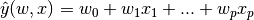## 1.1.1. 普通最小二乘法

LinearRegression 拟合一个带有系数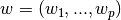的线性模型，使得数据集实际观测数据和预测数据（估计值）之间的残差平方和最小。其数学表达式为: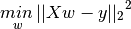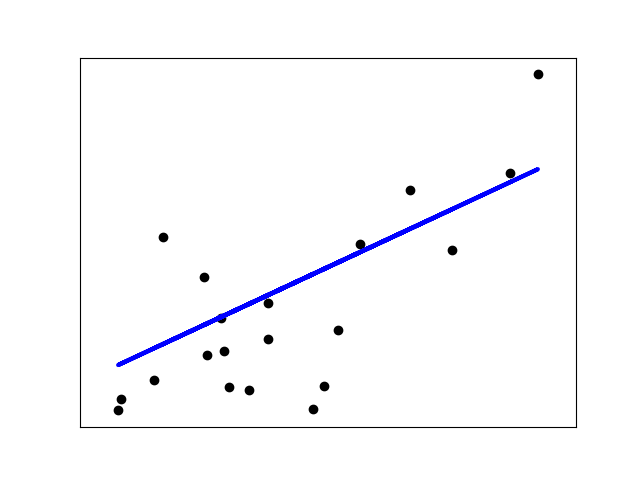LinearRegression 会调用 fit 方法来拟合数组 X， y，并且将线性模型的系数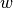存储在其成员变量 coef_ 中:

>>> from sklearn import linear_model
>>> reg = linear_model.LinearRegression()
>>> reg.fit ([[0, 0], [1, 1], [2, 2]], [0, 1, 2])
LinearRegression(copy_X=True, fit_intercept=True, n_jobs=1, normalize=False)
>>> reg.coef_
array([ 0.5,  0.5])



## 1.1.2. 岭回归

Ridge 回归通过对系数的大小施加惩罚来解决 普通最小二乘法 的一些问题。 岭系数最小化的是带罚项的残差平方和，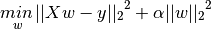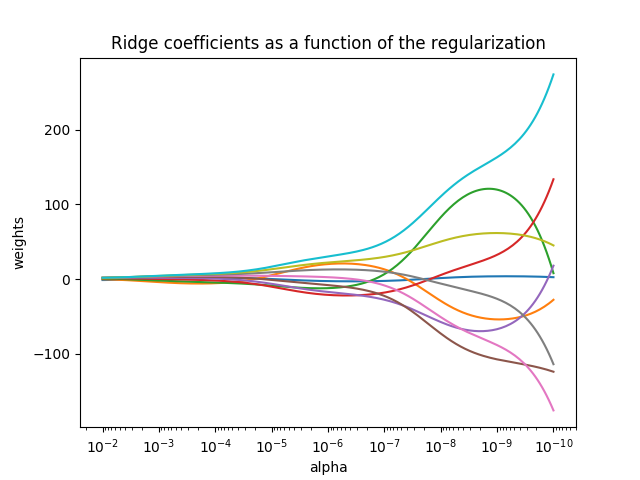>>> from sklearn import linear_model
>>> reg = linear_model.Ridge (alpha = .5)
>>> reg.fit ([[0, 0], [0, 0], [1, 1]], [0, .1, 1])
Ridge(alpha=0.5, copy_X=True, fit_intercept=True, max_iter=None,
normalize=False, random_state=None, solver='auto', tol=0.001)
>>> reg.coef_
array([ 0.34545455,  0.34545455])
>>> reg.intercept_
0.13636...



### 1.1.2.2. 设置正则化参数：广义交叉验证

RidgeCV 通过内置的关于的 alpha 参数的交叉验证来实现岭回归。 该对象与 GridSearchCV 的使用方法相同，只是它默认为 Generalized Cross-Validation(广义交叉验证 GCV)，这是一种有效的留一验证方法（LOO-CV）:

>>> from sklearn import linear_model
>>> reg = linear_model.RidgeCV(alphas=[0.1, 1.0, 10.0])
>>> reg.fit([[0, 0], [0, 0], [1, 1]], [0, .1, 1])
RidgeCV(alphas=[0.1, 1.0, 10.0], cv=None, fit_intercept=True, scoring=None,
normalize=False)
>>> reg.alpha_
0.1



## 1.1.3. Lasso

Lasso 是拟合稀疏系数的线性模型。 它在一些情况下是有用的，因为它倾向于使用具有较少参数值的情况，有效地减少给定解决方案所依赖变量的数量。 因此，Lasso 及其变体是压缩感知领域的基础。 在一定条件下，它可以恢复一组非零权重的精确集（见压缩感知_断层重建）。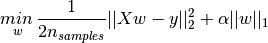lasso estimate 解决了加上罚项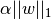的最小二乘法的最小化，其中，是一个常数，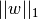是参数向量的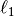-norm 范数。

Lasso 类的实现使用了 coordinate descent （坐标下降算法）来拟合系数。 查看 最小角回归 ，这是另一种方法:

>>> from sklearn import linear_model
>>> reg = linear_model.Lasso(alpha = 0.1)
>>> reg.fit([[0, 0], [1, 1]], [0, 1])
Lasso(alpha=0.1, copy_X=True, fit_intercept=True, max_iter=1000,
normalize=False, positive=False, precompute=False, random_state=None,
selection='cyclic', tol=0.0001, warm_start=False)
>>> reg.predict([[1, 1]])
array([ 0.8])



• “Regularization Path For Generalized linear Models by Coordinate Descent”, Friedman, Hastie & Tibshirani, J Stat Softw, 2010 (Paper).
• “An Interior-Point Method for Large-Scale L1-Regularized Least Squares,” S. J. Kim, K. Koh, M. Lustig, S. Boyd and D. Gorinevsky, in IEEE Journal of Selected Topics in Signal Processing, 2007 (Paper)

### 1.1.3.1. 设置正则化参数

alpha 参数控制估计系数的稀疏度。

#### 1.1.3.1.1. 使用交叉验证

scikit-learn 通过交叉验证来公开设置 Lasso alpha 参数的对象: LassoCVLassoLarsCVLassoLarsCV 是基于下面将要提到的 最小角回归 算法。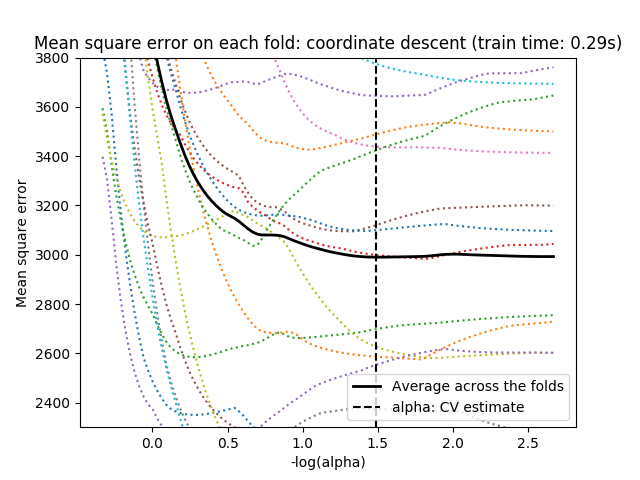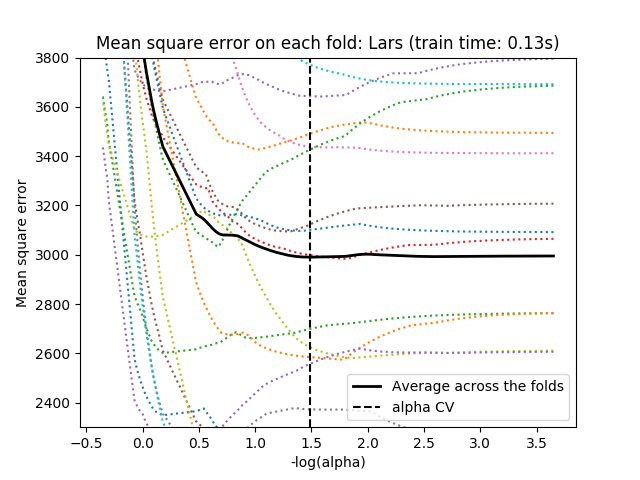#### 1.1.3.1.2. 基于信息标准的模型选择#### 1.1.3.1.3. 与 SVM 的正则化参数的比较

alpha 和 SVM 的正则化参数C 之间的等式关系是 alpha = 1 / C 或者 alpha = 1 / (n_samples * C) ，并依赖于估计器和模型优化的确切的目标函数。

## 1.1.4. 多任务 Lasso

MultiTaskLasso 是一个估计多元回归稀疏系数的线性模型： y 是一个形状为(n_samples, n_tasks) 的二维数组，其约束条件和其他回归问题（也称为任务）是一样的，都是所选的特征值。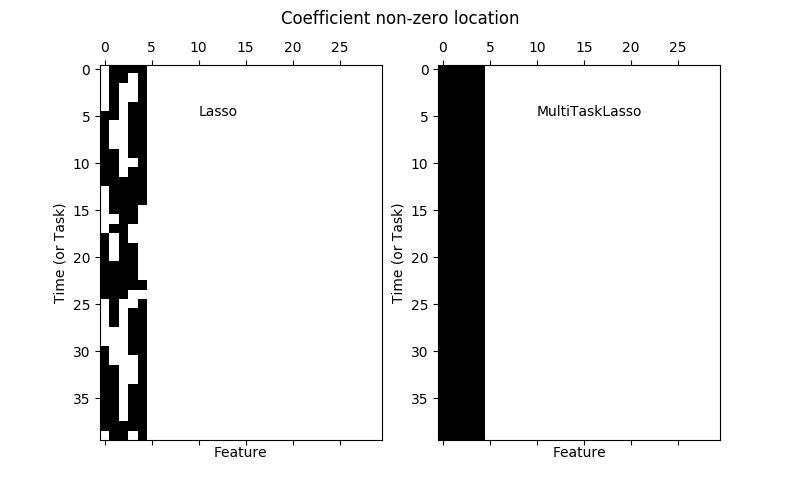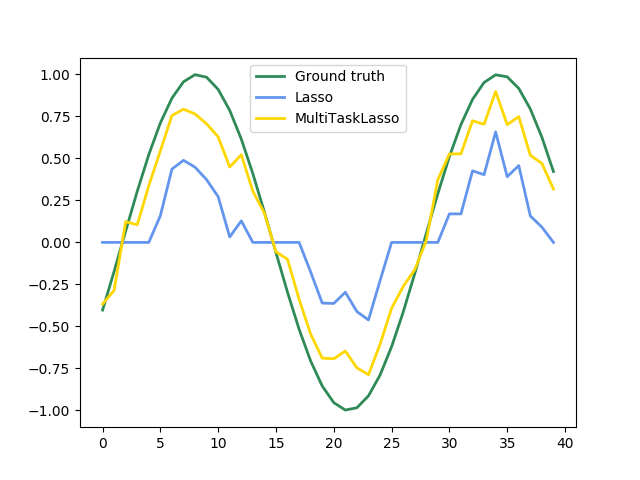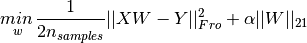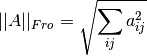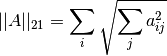MultiTaskLasso 类的实现使用了坐标下降作为拟合系数的算法。

## 1.1.5. 弹性网络

弹性网络 是一种使用 L1， L2 范数作为先验正则项训练的线性回归模型。 这种组合允许拟合到一个只有少量参数是非零稀疏的模型，就像 Lasso 一样，但是它仍然保持了一些类似于 Ridge 的正则性质。我们可利用 l1_ratio 参数控制 L1 和 L2 的凸组合。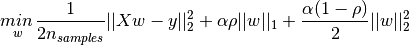ElasticNetCV 类可以通过交叉验证来设置参数 alpha） 和 l1_ratio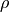） 。

• “Regularization Path For Generalized linear Models by Coordinate Descent”, Friedman, Hastie & Tibshirani, J Stat Softw, 2010 (Paper).
• “An Interior-Point Method for Large-Scale L1-Regularized Least Squares,” S. J. Kim, K. Koh, M. Lustig, S. Boyd and D. Gorinevsky, in IEEE Journal of Selected Topics in Signal Processing, 2007 (Paper)

## 1.1.6. 多任务弹性网络

MultiTaskElasticNet 是一个对多回归问题估算稀疏参数的弹性网络: Y 是一个二维数组，形状是 (n_samples,n_tasks)。 其限制条件是和其他回归问题一样，是选择的特征，也称为 tasks 。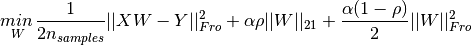MultiTaskElasticNet 类中的实现采用了坐标下降法求解参数。

MultiTaskElasticNetCV 中可以通过交叉验证来设置参数 alpha） 和 l1_ratio） 。

## 1.1.7. 最小角回归

LARS的优点:

• 当 p >> n，该算法数值运算上非常有效。(例如当维度的数目远超点的个数)
• 它在计算上和前向选择一样快，和普通最小二乘法有相同的运算复杂度。
• 它产生了一个完整的分段线性的解决路径，在交叉验证或者其他相似的微调模型的方法上非常有用。
• 如果两个变量对响应几乎有相等的联系，则它们的系数应该有相似的增长率。因此这个算法和我们直觉 上的判断一样，而且还更加稳定。
• 它很容易修改并为其他估算器生成解，比如Lasso。

LARS 的缺点:

• 因为 LARS 是建立在循环拟合剩余变量上的，所以它对噪声非常敏感。这个问题，在 2004 年统计年鉴的文章由 Weisberg 详细讨论。

LARS 模型可以在 Lars ，或者它的底层实现 lars_pathlars_path_gram中被使用。

## 1.1.8. LARS Lasso

LassoLars 是一个使用 LARS 算法的 lasso 模型，不同于基于坐标下降法的实现，它可以得到一个精确解，也就是一个关于自身参数标准化后的一个分段线性解。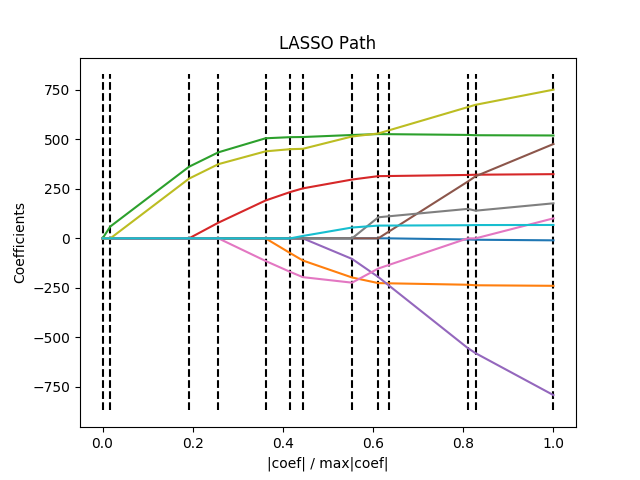>>> from sklearn import linear_model
>>> reg = linear_model.LassoLars(alpha=.1)
>>> reg.fit([[0, 0], [1, 1]], [0, 1])
LassoLars(alpha=0.1, copy_X=True, eps=..., fit_intercept=True,
fit_path=True, max_iter=500, normalize=True, positive=False,
precompute='auto', verbose=False)
>>> reg.coef_
array([0.717157..., 0.        ])



Lars 算法提供了一个几乎无代价的沿着正则化参数的系数的完整路径，因此常利用函数 lars_pathlars_path_gram来取回路径。

## 1.1.9. 正交匹配追踪法（OMP）

OrthogonalMatchingPursuit (正交匹配追踪法)和 orthogonal_mp使用了 OMP 算法近似拟合了一个带限制的线性模型，该限制影响于模型的非 0 系数(例：L0 范数)。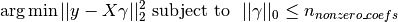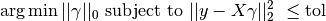OMP 是基于每一步的贪心算法，其每一步元素都是与当前残差高度相关的。它跟较为简单的匹配追踪（MP）很相似，但是相比 MP 更好，在每一次迭代中，可以利用正交投影到之前选择的字典元素重新计算残差。

## 1.1.10. 贝叶斯回归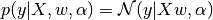Alpha 在这里也是作为一个变量，通过数据中估计得到。

• 它能根据已有的数据进行改变。
• 它能在估计过程中引入正则项。

• 它的推断过程是非常耗时的。

### 1.1.10.1. 贝叶斯岭回归

BayesianRidge 利用概率模型估算了上述的回归问题，其先验参数是由以下球面高斯公式得出的：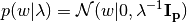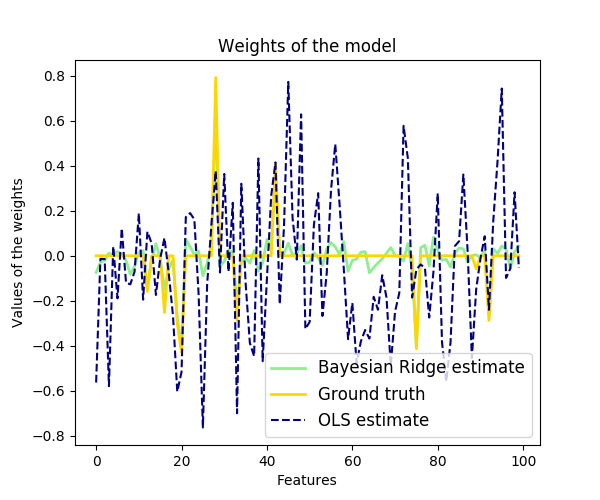>>> from sklearn import linear_model
>>> X = [[0., 0.], [1., 1.], [2., 2.], [3., 3.]]
>>> Y = [0., 1., 2., 3.]
>>> reg = linear_model.BayesianRidge()
>>> reg.fit(X, Y)
BayesianRidge(alpha_1=1e-06, alpha_2=1e-06, compute_score=False, copy_X=True,
fit_intercept=True, lambda_1=1e-06, lambda_2=1e-06, n_iter=300,
normalize=False, tol=0.001, verbose=False)



>>> reg.predict ([[1, 0.]])
array([ 0.50000013])



>>> reg.coef_
array([ 0.49999993,  0.49999993])



### 1.1.10.2. 主动相关决策理论 - ARD

ARDRegression （主动相关决策理论）和 Bayesian Ridge Regression 非常相似，但是会导致一个更加稀疏的权重w

ARDRegression 提出了一个不同的的先验假设。具体来说，就是弱化了高斯分布为球形的假设。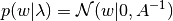Bayesian Ridge Regression_ 不同， 每个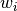都有一个标准差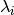。所有的先验分布 由超参数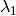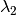确定的相同的 gamma 分布确定。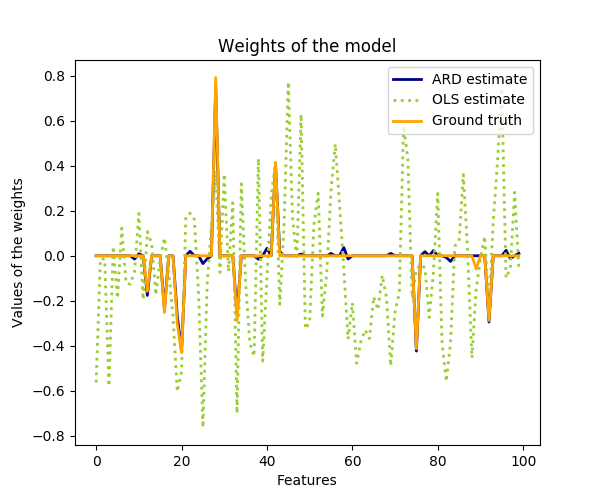ARD 也被称为 稀疏贝叶斯学习相关向量机 。

## 1.1.11. logistic 回归

logistic 回归，虽然名字里有 “回归” 二字，但实际上是解决分类问题的一类线性模型。在某些文献中，logistic 回归又被称作 logit 回归，maximum-entropy classification（MaxEnt，最大熵分类），或 log-linear classifier（对数线性分类器）。该模型利用函数 logistic function 将单次试验（single trial）的可能结果输出为概率。

scikit-learn 中 logistic 回归在 LogisticRegression 类中实现了二分类（binary）、一对多分类（one-vs-rest）及多项式 logistic 回归，并带有可选的 L1 和 L2 正则化。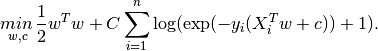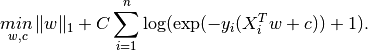Elastic-Net正则化是L1 和 L2的组合，来使如下代价函数最小: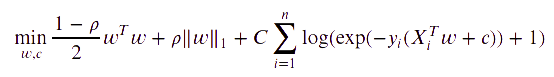LogisticRegression 类中实现了这些优化算法: liblinearnewton-cglbfgssagsaga

liblinear应用了坐标下降算法（Coordinate Descent, CD），并基于 scikit-learn 内附的高性能 C++ 库 LIBLINEAR library 实现。不过 CD 算法训练的模型不是真正意义上的多分类模型，而是基于 “one-vs-rest” 思想分解了这个优化问题，为每个类别都训练了一个二元分类器。因为实现在底层使用该求解器的 LogisticRegression 实例对象表面上看是一个多元分类器。 sklearn.svm.l1_min_c 可以计算使用 L1时 C 的下界，以避免模型为空（即全部特征分量的权重为零）。

lbfgs, sagnewton-cg 求解器只支持 L2罚项以及无罚项，对某些高维数据收敛更快。这些求解器的参数 multi_class设为 multinomial 即可训练一个真正的多项式 logistic 回归  ，其预测的概率比默认的 “one-vs-rest” 设定更为准确。

sag 求解器基于平均随机梯度下降算法（Stochastic Average Gradient descent） 。在大数据集上的表现更快，大数据集指样本量大且特征数多。

saga 求解器  是 sag 的一类变体，它支持非平滑（non-smooth）的 L1 正则选项 penalty="l1" 。因此对于稀疏多项式 logistic 回归 ，往往选用该求解器。saga求解器是唯一支持弹性网络正则选项的求解器。

lbfgs是一种近似于Broyden–Fletcher–Goldfarb–Shanno算法的优化算法，属于准牛顿法。lbfgs求解器推荐用于较小的数据集，对于较大的数据集，它的性能会受到影响。

liblinear 的区别:

fit_intercept=False 拟合得到的 coef_ 或者待预测的数据为零时，用 solver=liblinearLogisticRegressionLinearSVC 与直接使用外部 liblinear 库预测得分会有差异。这是因为， 对于 decision_function 为零的样本， LogisticRegressionLinearSVC 将预测为负类，而 liblinear 预测为正类。 注意，设定了 fit_intercept=False ，又有很多样本使得 decision_function 为零的模型，很可能会欠拟合，其表现往往比较差。建议您设置 fit_intercept=True 并增大 intercept_scaling

LogisticRegressionCV 对 logistic 回归 的实现内置了交叉验证（cross-validation），可以找出最优的 Cl1_ratio参数 。newton-cgsagsagalbfgs 在高维数据上更快，这是因为采用了热启动（warm-starting）。

## 1.1.12. 随机梯度下降， SGD

SGDClassifierSGDRegressor 分别用于拟合分类问题和回归问题的线性模型，可使用不同的（凸）损失函数，支持不同的罚项。 例如，设定 loss="log" ，则 SGDClassifier 拟合一个逻辑斯蒂回归模型，而 loss="hinge" 拟合线性支持向量机（SVM）。

## 1.1.13. Perceptron（感知器）

Perceptron 是适用于大规模学习的一种简单算法。默认情况下：

• 不需要设置学习率（learning rate）。
• 不需要正则化处理。
• 仅使用错误样本更新模型。

## 1.1.15. 稳健回归（Robustness regression）: 处理离群点（outliers）和模型错误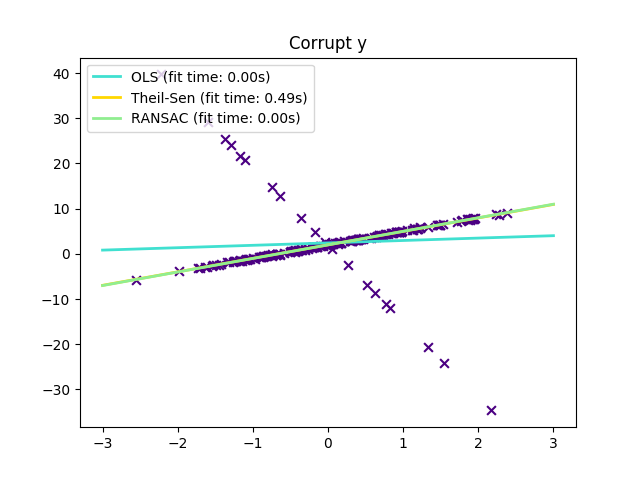### 1.1.15.1. 各种使用场景与相关概念

• HuberRegressor 一般快于 RANSACTheil Sen ，除非样本数很大，即 n_samples >> n_features 。 这是因为 RANSACTheil Sen 都是基于数据的较小子集进行拟合。但使用默认参数时， Theil SenRANSAC 可能不如 HuberRegressor 鲁棒。
• RANSACTheil Sen 更快，在样本数量上的伸缩性（适应性）更好。
• RANSAC 能更好地处理y方向的大值离群点（通常情况下）。
• Theil Sen 能更好地处理x方向中等大小的离群点，但在高维情况下无法保证这一特点。 实在决定不了的话，请使用 RANSAC

### 1.1.15.2. RANSAC： 随机抽样一致性算法（RANdom SAmple Consensus）

RANSAC 是一种非确定性算法，以一定概率输出一个可能的合理结果，依赖于迭代次数（参数 max_trials）。这种算法主要解决线性或非线性回归问题，在计算机视觉摄影测绘领域尤为流行。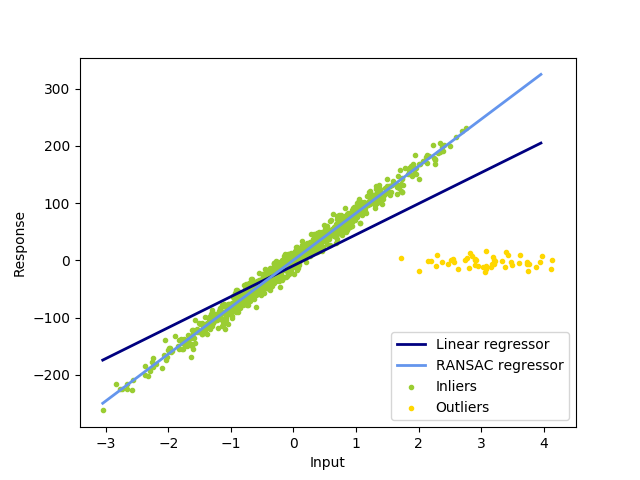#### 1.1.15.2.1. 算法细节

1. 从原始数据中抽样 min_samples 数量的随机样本，检查数据是否合法（见 is_data_valid ）。
2. 用一个随机子集拟合模型（ base_estimator.fit ）。检查模型是否合法（见 is_model_valid ）。
3. 计算预测模型的残差（residual），将全体数据分成局内点和离群点（ base_estimator.predict(X) - y ）。绝对残差小于 residual_threshold 的全体数据认为是局内点。
4. 若局内点样本数最大，保存当前模型为最佳模型。以免当前模型离群点数量恰好相等（而出现未定义情况），规定仅当数值大于当前最值时认为是最佳模型。

### 1.1.15.3. Theil-Sen 预估器: 广义中值估计器（generalized-median-based estimator）

TheilSenRegressor 估计器：使用中位数在多个维度泛化，对多元异常值更具有鲁棒性，但问题是，随着维数的增加，估计器的准确性在迅速下降。准确性的丢失，导致在高维上的估计值比不上普通的最小二乘法。

#### 1.1.15.3.1. 算法理论细节

TheilSenRegressor 在渐近效率和无偏估计方面足以媲美 Ordinary Least Squares (OLS) （普通最小二乘法（OLS））。与 OLS 不同的是， Theil-Sen 是一种非参数方法，这意味着它没有对底层数据的分布假设。由于 Theil-Sen 是基于中值的估计，它更适合于损坏的数据即离群值。 在单变量的设置中，Theil-Sen 在简单的线性回归的情况下，其崩溃点大约 29.3% ，这意味着它可以容忍任意损坏的数据高达 29.3% 。scikit-learn 中实现的 TheilSenRegressor 是多元线性回归模型的推广  ，利用了空间中值方法，它是多维中值的推广### 1.1.15.4. Huber 回归

HuberRegressorRidge 不同，因为它对于被分为异常值的样本应用了一个线性损失。如果这个样品的绝对误差小于某一阈值，样品就被分为内围值。 它不同于 TheilSenRegressorRANSACRegressor ，因为它没有忽略异常值的影响，并分配给它们较小的权重。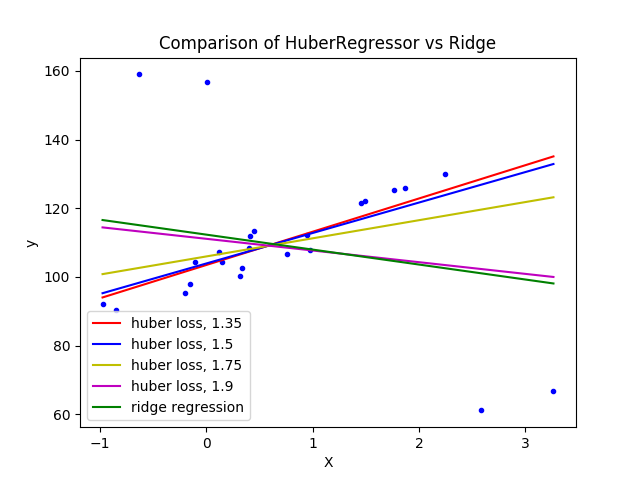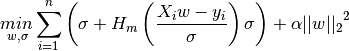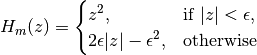### 1.1.15.5. 注意

HuberRegressor 与将损失设置为 huberSGDRegressor 并不相同，体现在以下方面的使用方式上。

• HuberRegressor 是标度不变性的. 一旦设置了 epsilon ， 通过不同的值向上或向下缩放 Xy ，就会跟以前一样对异常值产生同样的鲁棒性。相比 SGDRegressor 其中 epsilonXy 被缩放的时候必须再次设置。
• HuberRegressor 应该更有效地使用在小样本数据，同时 SGDRegressor 需要一些训练数据的 passes 来产生一致的鲁棒性。

## 1.1.16. 多项式回归：用基函数展开线性模型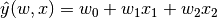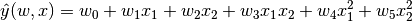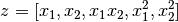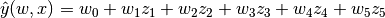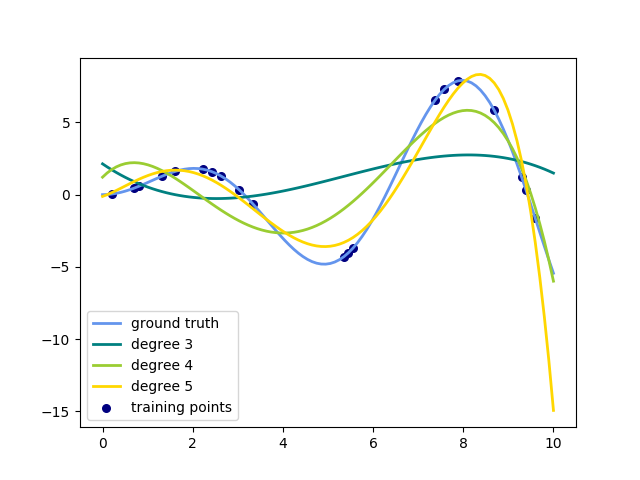>>> from sklearn.preprocessing import PolynomialFeatures
>>> import numpy as np
>>> X = np.arange(6).reshape(3, 2)
>>> X
array([[0, 1],
[2, 3],
[4, 5]])
>>> poly = PolynomialFeatures(degree=2)
>>> poly.fit_transform(X)
array([[  1.,   0.,   1.,   0.,   0.,   1.],
[  1.,   2.,   3.,   4.,   6.,   9.],
[  1.,   4.,   5.,  16.,  20.,  25.]])



X 的特征已经从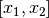转换到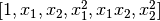, 并且现在可以用在任何线性模型。

>>> from sklearn.preprocessing import PolynomialFeatures
>>> from sklearn.linear_model import LinearRegression
>>> from sklearn.pipeline import Pipeline
>>> import numpy as np
>>> model = Pipeline([('poly', PolynomialFeatures(degree=3)),
...                   ('linear', LinearRegression(fit_intercept=False))])
>>> # fit to an order-3 polynomial data
>>> x = np.arange(5)
>>> y = 3 - 2 * x + x ** 2 - x ** 3
>>> model = model.fit(x[:, np.newaxis], y)
>>> model.named_steps['linear'].coef_
array([ 3., -2.,  1., -1.])



>>> from sklearn.linear_model import Perceptron
>>> from sklearn.preprocessing import PolynomialFeatures
>>> import numpy as np
>>> X = np.array([[0, 0], [0, 1], [1, 0], [1, 1]])
>>> y = X[:, 0] ^ X[:, 1]
>>> y
array([0, 1, 1, 0])
>>> X = PolynomialFeatures(interaction_only=True).fit_transform(X).astype(int)
>>> X
array([[1, 0, 0, 0],
[1, 0, 1, 0],
[1, 1, 0, 0],
[1, 1, 1, 1]])
>>> clf = Perceptron(fit_intercept=False, max_iter=10, tol=None,
...                  shuffle=False).fit(X, y)



>>> clf.predict(X)
array([0, 1, 1, 0])
>>> clf.score(X, y)
1.0



{% endraw %}